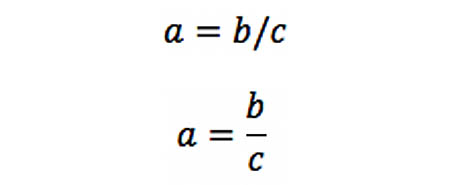# Definition: formula editor

Software that enables the creation of mathematical formulas. Formula editors may be part of a word processing program or stand-alone applications that enable formulas to be created and inserted into word processing documents. Such programs may also be able to create an image of the formula, which is expressed as a bitmap without the possibility of future editing. See bitmap.Equation Support
Word for Mac Version 16 has basic support for equations. In this example, when a = b divided by c is entered (top), it is automatically converted into the equation format (bottom).